Published: 15 August 2017

# Prediction of natural frequency of basalt fiber reinforced polymer (FRP) laminated variable thickness plates with intermediate elastic support using artificial neural networks (ANNs) method

Wael A. Altabey1
1International Institute for Urban Systems Engineering, Southeast University, Nanjing, 210096, China
1Department of Mechanical Engineering, Faculty of Engineering, Alexandria University, Alexandria, 21544, Egypt
Views 132

#### Abstract

The paper is focused on the application of artificial neural networks (ANNs) in predicting the natural frequency of basalt fiber reinforced polymer (FRP) laminated, variable thickness plates. The author has found that the finite strip transition matrix (FSTM) approach is very effective to study the changes of plate natural frequencies due to intermediate elastic support (IES), but the method difficulty in terms of, a lot of calculations with large number of iterations is the main drawback of the method. For training and testing of the ANN model, a number of FSTM results for different classical boundary conditions (CBCs) with different values of elastic restraint coefficients (${K}_{T}$) for IES have been carried out to training and testing an ANN model. The ANN model has been developed using multilayer perceptron (MLP) Feed-forward neural networks (FFNN). The adequacy of the developed model is verified by the regression coefficient (${R}^{2}$) and Mean Square error (MSE) It was found that the R2 and MSE values are 0.986 and 0.0134 for train and 0.9966 and 0.0122 for test data respectively. The results showed that, the training algorithm of FFNN was sufficient enough in predicting the natural frequency in basalt FRP laminated, variable thickness plates with IES. To judge the ability and efficiency of the developed ANN model, MSE has been used. The results predicted by ANN are in very good agreement with the FSTM results. Consequently, the ANN is show to be effective in predicting the natural frequency of laminated composite plates.

## 1. Introduction

Continuous laminated plates and laminated composite plates with intermediate stiffeners are one of very common in composite structures that used in many engineering fields such as aerospace, civil and marine industries.

Natural frequencies of laminated composite structures are the classical tools for the intelligent diagnosis of composite defects (e.g. fiber breakage, matrix cracks, de-bonding and delamination). Vibration response has become an important tool in Structural health monitoring (SHM) in the last decades, the natural frequencies of composite structure are extracted and analyzed under each damage scenario as damage detection and localization technique based on the changes in vibration parameters. In the recent years, several approaches to find the natural frequencies and the mode shapes of laminated composite plates became a field that has attracted a lot of interest in the scientific community.

In order to find the natural frequencies and the mode shapes for different boundary conditions with IES, a numerical approach or an approximate method must be used. Several researchers are attracted to vibration of plates with IES. FSTM is one of a semi-analytical methods are welcomed in the many literatures as an alternative to the exact solution. In our previous paper by Altabey , a semi-analytical method, the FSTM approach has been used to investigate the free vibration of basalt FRP laminated variable thickness rectangular plates with IES. Since all of the coefficients in the FSTM method can be obtained, a lot of calculations with large number of iterations must be performed to obtain a frequency parameters, i.e. the time of solutions will be increased, and the large amount of data are required to study the changes of plate natural frequencies due to IES. This is the main drawback of the method identified so far.

In the present study, the laminated composite plate shown in Fig. 1 has been modeled and analyzed using FSTM method. The laminated composite plate was manufactured using five symmetrically, angle-ply, laminates with the fiber orientations [45º/-45º/45º/-45º/45º] of basalt fiber and a polymer resin matrix. A predictive model for natural frequency in terms of fiber orientations is then developed using artificial neural networks. The developed model is tested with the FSTM data which were never used for developing the model. The FSTM results show that R2 and MSE are 0.986 and 0.0134 for train and 0.9966 and 0.0122 for test data respectively. Hence ANN model predicted results are in very good agreement with the FSTM results. Consequently, ANN are shown to be very effective in predicting the natural frequency of laminated composite plates under Four different CBCs are used in the analysis with different KT for IES.

Fig. 1The geometrical model of Basalt FRP laminated variable thickness rectangular plate with IES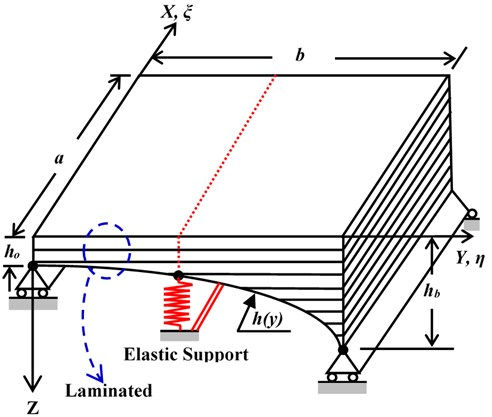## 2. Governing Equations

The normalized partial differential equation governing the vibration of symmetrically, angle-ply laminated, variable thickness, rectangular plates under the assumption of the classical deformation theory in terms of the plate deflection ${w}_{o}\left(x,y,t\right)$ using the non-Dimensional variables $\xi$ and $\eta$ is given by [1, 2]:

1
$\begin{array}{l}{\psi }_{1}\frac{1}{{a}^{4}}{W}_{\xi \xi \xi \xi }+\frac{2{\psi }_{2}}{{h}^{3}\left(\eta \right)}\frac{1}{{a}^{2}b}\frac{\partial {h}^{3}\left(\eta \right)}{\partial \eta }{W}_{\xi \xi \eta }+2{\psi }_{2}\frac{1}{{a}^{2}{b}^{2}}{W}_{\xi \xi \eta \eta }+{\psi }_{3}\frac{1}{{a}^{3}b}{W}_{\xi \xi \xi \eta }\\ +4{\psi }_{4}\frac{1}{a{b}^{3}}{W}_{\xi \eta \eta \eta }+\frac{1}{ab}\frac{4{\psi }_{4}}{{h}^{3}\left(\eta \right)}\frac{{\partial }^{2}{h}^{3}\left(\eta \right)}{\partial {\eta }^{2}}{W}_{\xi \eta }+\frac{8{\psi }_{4}}{{h}^{3}\left(\eta \right)}\frac{1}{a{b}^{2}}\frac{\partial {h}^{3}\left(\eta \right)}{\partial \eta }{W}_{\xi \eta \eta }\\ +\frac{1}{{b}^{2}}\frac{1}{{h}^{3}\left(\eta \right)}\frac{{\partial }^{2}{h}^{3}\left(\eta \right)}{\partial {\eta }^{2}}{W}_{\eta \eta }+\frac{1}{{b}^{4}}{W}_{\eta \eta \eta \eta }+\frac{2}{{h}^{3}\left(\eta \right)}\frac{1}{{b}^{3}}\frac{\partial {h}^{3}\left(\eta \right)}{\partial \eta }{W}_{\eta \eta \eta }=-\frac{{m}_{o}}{{D}_{22}}\frac{{h}_{o}^{2}}{{h}^{2}\left(\eta \right)}{W}_{tt},\end{array}$

where $\beta =a/b$ is the aspect ratio, also:

$\xi =\frac{x}{a},\eta =\frac{y}{b},{\psi }_{1}=\frac{{D}_{11}}{{D}_{22}},{\psi }_{2}=\frac{\left({D}_{12}+2{D}_{66}\right)}{{D}_{22}},{\psi }_{3}=\frac{{D}_{16}}{{D}_{22}},{\psi }_{4}=\frac{{D}_{26}}{{D}_{22}},$
${W}_{\xi \xi \xi \xi }=\frac{{\partial }^{4}{w}_{o}}{\partial {\xi }^{4}},{W}_{\eta \eta \eta \eta }=\frac{{\partial }^{4}{w}_{o}}{\partial {\eta }^{4}},{W}_{\xi \xi \eta \eta }=\frac{{\partial }^{4}{w}_{o}}{\partial {\xi }^{2}\partial {\eta }^{2}},$
${W}_{\xi \xi \xi \eta }=\frac{{\partial }^{4}{w}_{o}}{\partial {\xi }^{3}\partial \eta },{W}_{\eta \eta \eta \xi }=\frac{{\partial }^{4}{w}_{o}}{\partial {\eta }^{3}\partial \xi },{W}_{\eta \eta }=\frac{{\partial }^{2}{w}_{o}}{\partial {t}^{2}},{m}_{o}=\rho {h}_{o}$

${D}_{ij}$ is the flexural rigidities matrix of the plate.

Since the treatment of IES conditions are the main objective of this paper we presented it in more details. At IES, $y=b/2$, the displacement must vanish and the moment must be continuous, i.e. :

2
$\frac{1}{b}{\frac{\partial {w}_{0}}{\partial \eta }|}_{\eta ={1}^{-}/2}=\frac{1}{b}{\frac{\partial {w}_{0}}{\partial \eta }|}_{\eta ={1}^{+}/2}$

## 3. Finite strip transition matrix (FSTM) with artificial neural network (ANN) method

### 3.1. Finite strip transition matrix (FSTM) method

The method is made when such a shape function is not conveniently obtained in case of discussing the plate problems by series. The plate may be divided into N discrete longitudinal strips spanning between supports as shown in Fig. 2. By basic displacement interpolation functions may then be used to represent displacement field within and between individual strips.

For a plate striped in the $\xi$-direction as shown in Fig. 2, the shape function $W\left(\xi ,\eta ,t\right)$ may be assumed in the form:

4
$W\left(\xi ,\eta ,t\right)={\sum }_{i=0}^{N}{X}_{i}\left(\xi \right){Y}_{i}\left(\eta \right){e}^{i\omega t},$

where: ${Y}_{i}\left(\eta \right)$ is unknown function to be determined and ${X}_{i}\left(\xi \right)$ is chosen a priori, the basic function in $\xi$-direction.

Fig. 2Finite strip simulation on plate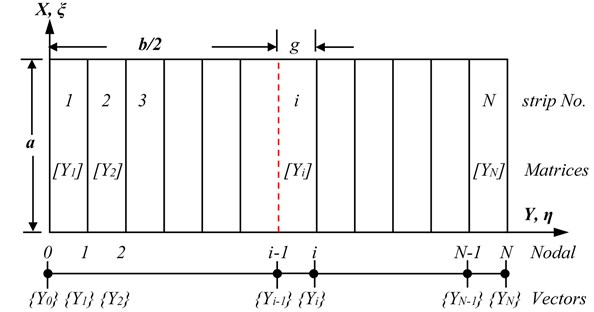### 3.2. Artificial neural network (ANN) modeling

Artificial neural network (ANN) is an attractive inductive approach for modeling non-linear and complex systems without explicit physical representation and thus provides an alternative approach for modeling hydrologic systems. Artificial neural network was first developed in the 1940s. Generally speaking, ANNs are information processing systems. In recent decades, considerable interest has been raised over their practical applications. Training of artificial neural network enables the system to capture the complex and non-linear relationships that are not easily analyzed by using conventional methods such as linear and multiple regression methods and the network is built directly from experimental or numerical data by its self-organizing capabilities. Based on the different applications, various types of neural network with various algorithms have been employed to solve the different problems. In this work will be using the preferred ANN structures to predict the frequency parameter of presented numerical conditions.

### 3.2.1. ANN configuration

Since the ANN configuration has a great influence on the predictive quality, various arrangements have been considered in previous work. It is necessary to define a simple code to describe the ANN configuration, as follows:

5
$\left\{{N}_{in}{\left[\begin{array}{ll}{N}_{h1}& {N}_{h2}\end{array}\right]}_{e}{N}_{out}\right\},$

where ${N}_{in}$ and ${N}_{out}$ are the element numbers of input and output parameters, respectively, and $e$ is the number of hidden layers. ${N}_{h1}$ and ${N}_{h2}$ are numbers of neurons in each hidden layer, respectively. For example, $\left\{7{\left[21\right]}_{1}1\right\}$ means a one hidden layer ANN with seven input and one output parameters, with the hidden layer containing 21 elements (neurons); $\left\{9{\left[\begin{array}{ll}15& 10\end{array}\right]}_{2}1\right\}$ denotes a nine input and one output ANN, with 15 and 10 neurons, respectively, in two hidden layers.

The powerful function of an ANN is due to the neurons within the hidden layers, as well as to the related interconnections. Networks are also sensitive to the number of neurons in their hidden layers. It is believed that an ANN can represent any reasonable relationship between input and output if the hidden layers have enough neurons. However, for the practical case, more hidden neurons bring more interconnections, which require, in turn, larger training datasets for learning the relationships. It is therefore always necessary to optimize the number of neurons of the ANN hidden layers, as demonstrated by Demuth and Beale .

### 3.2.2. Performance evaluation measures

It is very useful from the designer point of view to have a neural system aids to decide whether his suggested design is suitable or not by Compute the MSE from equation:

6
$MSE=\sum \frac{{\left({\left(\mathrm{\Omega }\right)}_{nn}-\mathrm{\Omega }\right)}^{2}}{n},$

where: ${\left(\mathrm{\Omega }\right)}_{nn}$ is the predicted frequency parameter from ANN, $\mathrm{\Omega }$ is the target or computed values from FSTM method of frequency parameter, and $n$ is the number of FSTM computed data values.

Thus, the performance index will either have one global minimum, depending on the characteristics of the input vectors. Local minimum is the minimum of a function over a limited range of input values. Local minimum is an unavoidable when the ANN is fitted. So, a local minimum may be good or bad depending on how close the local minimum is to the global minimum and how low an MSE is required. In any case, the method applied to solve this problem and descent the local minimum with momentum. Momentum allows a network to respond not only to the local gradient, but also to recent trends in the error surface. Without momentum a network may get stuck in a shallow local minimum.

The estimation performances of frequency parameter $\left(\mathrm{\Omega }\right)$ is evaluated by the lack of fit with the regression coefficient of the multiple determination ${R}^{2}$; ${R}^{2}$ is defined as:

7
${R}^{2}=1-\frac{{\sum }_{n}{\left({\left(\mathrm{\Omega }\right)}_{nn}-\mathrm{\Omega }\right)}^{2}}{{\sum }_{n}{\left(\mathrm{\Omega }\right)}^{2}}.$

The value of ${R}^{2}$ is equal to or lower than 1.0. A higher value of ${R}^{2}$ implies a better fit. When the ANN shows a very good fit, ${R}^{2}$ approaches 1.0. A good fit of the ANN means that the ANN gives good estimations for the dielectric properties change used for the regression. Lower ${R}^{2}$ values means poorer estimations and the error band of the estimated result is wider.

### 3.3. Feed-forward neural networks (FFNN)

In this work we will use the suggested feed forward neural network, FFNN, to predict natural frequency of basalt FRP composite plate, because it has minimum mean square error (MSE), in composite structure applications [4, 5]. FFNN in general consist of a layer of input neurons, a layer of output neurons and one or more layers of hidden neurons . Neurons in each layer are interconnected fully to previous and next layer neurons with each interconnection have associated connection strength or weight. The activation function used in the hidden and output layers neurons is nonlinear, where as for the input layer no activation function is used since no computation is involved in that layer. Information flows from one layer to the other layer in a feed-forward manner. Various functions are used to model the neuron activity such as liner transfer function ($purelin\left(n\right)$), Tan-Sigmoid transfer function ($\mathrm{t}\mathrm{a}\mathrm{n}\mathrm{s}\mathrm{i}\mathrm{g}\left(n\right)$) or Radial Basis (Gaussian) transfer functions ($radbas\left(n\right)$).

The training process is terminated either when the (MSE) between the observed data and the ANN outcomes for all elements in the training set has reached a pre-specified threshold or after the completion of a pre-specified number of learning epochs.

## 4. Results and discussion

In this work, The FSTM with ANNs techniques are used to predict the free vibration of the laminated composite plate shown in Fig. 1. The plate was manufactured using five symmetrically, angle-ply, laminates with the fiber orientations [$\theta$/$-\theta$/$\theta$/$-\theta$/$\theta$] is [45°/–45°/45°/–45°/45°] of basalt fiber and a polymer resin matrix. Physical and mechanical properties of the basalt FRP laminate composite plate are shown in Table 1.

Table 1Physical and mechanical properties of the basalt FRP

 ${E}_{1}$ ${E}_{2}={E}_{3}$ ${G}_{1}={G}_{3}$ ${G}_{2}$ ${\upsilon }_{1}={\upsilon }_{3}$ ${\upsilon }_{2}$ $\rho$ 96.74 GPa 22.55 GPa 10.64 GPa 8.73GPa 0.3 0.6 2700 kg/m3

The non-dimensional frequency parameter $\mathrm{\Omega }$ are addressed in form :

8
$\mathrm{\Omega }=\sqrt{\frac{{m}_{o}h\left(\eta \right){\omega }^{2}{a}^{4}}{{h}_{o}{D}_{22}}}.$

The plate has linear deformation in thickness $h\left(y\right)$ woth non-dimensional form :

9
$h\left(\eta \right)=1+\mathrm{\Delta }\eta ,$

where: $\mathrm{\Delta }$ is the tapered ratio of plate given by $\mathrm{\Delta }={\left(h}_{b}-{h}_{o}\right)/{h}_{o}$, (${h}_{o}$) is the thickness of the plate at $\eta =$0 and (${h}_{b}$) is the thickness of the plate at $\eta =$1.

### 4.1. Convergence study and accuracy

In this subsection, the author has carried out for convergening the proposed method, first six frequencies are calculated and compared with available results in literatures . He compared the computational results of FSTM method from previous work  with values available from literatures [7, 8] for E-glass/ epoxy, square ($\beta =$1.0), uniform thickness ($\mathrm{\Delta }=$0) plates with elastic foundation support, the mechanical properties of the plate material are ${\upsilon }_{1}=$${\upsilon }_{2}=$ 0.23, $D=E{h}^{3}/\left[12\left(1-{\upsilon }^{2}\right)\right]$, and ${D}_{66}=\left(1-\upsilon \right)D/2$. Two different CBCs (SSSS and CCCC) are used in calculations, KT is taken equal to 500, 1390.2 for SSSS and CCCC respectively. In this study are addressed in form $\mathrm{\Omega }={\left(\rho h{\omega }^{2}{a}^{4}/D\right)}^{1/2}$. He found the results by FSTM method are in a very close agreement with results in References [7, 8] and the convergence speed can be achieved with terms of series solution ($N=$ 3 to 7).

### 4.2. Finite strip transition matrix (FSTM) results

In the present study, the numerical computations using FSTM approach is applied with an ANNs, which are combined to decrease detection effort to discern for different KT by minimizing the number of FSTM computations in order to keep save the time of calculations to a minimum. The method has successfully computations of the frequency parameter using only two different ${K}_{T}$, the first one is located at ${K}_{T}=$ 50 the second is ${K}_{T}=$ 750, respectively, as shown in Table 2.

Table 2 presents the first six frequencies of the laminated composite plate shown in Fig. 1. The plate has the parameters of aspect ratio ($\beta$) and thickness tapered ratio ($\Delta$) are 0.5. The Four different type of CBCs (SSSS, CCCC, SSFF and CCFF) and two different values of KT of IES are used in the calculations to study the changes of natural frequencies due to IES. The locations of the IES is at mid-line of the presented plate.

Table 2The first six frequencies of laminated, plate shown in Fig. 1 for two different values of KT, (Δ=0.5), (β=0.5)

 ${K}_{T}$ ${\mathrm{\Omega }}_{1}$ ${\mathrm{\Omega }}_{2}$ ${\mathrm{\Omega }}_{3}$ ${\mathrm{\Omega }}_{4}$ ${\mathrm{\Omega }}_{5}$ ${\mathrm{\Omega }}_{6}$ SSSS 50 22.1450 36.2210 53.5870 78.2360 105.5870 138.6970 750 55.0692 69.1452 86.5112 111.1602 138.5112 171.6212 CCCC 50 28.4310 46.5025 68.7979 100.4437 135.5584 178.0668 750 61.3551 79.4267 101.7221 133.3678 168.4826 210.9910 SSFF 50 12.2750 20.0773 29.7033 43.3663 58.5270 76.8799 750 45.1992 53.0015 62.6275 76.2905 91.4511 109.8041 CCFF 50 19.5928 32.0466 47.4112 69.2194 93.4182 122.7123 750 52.5170 64.9707 80.3353 102.1436 126.3424 155.6365

### 4.3. A feed-forward neural network (FFNN) design for predicting frequency parameters

A FFNN configuration in this case was $\left\{2{\left[\begin{array}{ll}5& 5\end{array}\right]}_{2}1\right\}$, with tan-sigmoid neurons for the first layer while the second layer has pure linear ones. FFNN is trained by measuring values of ${K}_{T}$, $\theta$ to predict $\mathrm{\Omega }$ for four different CBCs are SSSS, CCCC, SSFF and CCFF. Determination of the hidden layer, in addition to the number of nodes in the input and output layers, for providing the best training results, was the initial phase of the training procedure. The target for MSE to be reached at the end of the simulations was 0.001. Since the second step was largely a trial-and-error process, and involved FFNNs with the number of hidden layer neurons more than five, it did not show any sizeable improvement in prediction accuracy. Thus, the number of neurons for the single hidden layer was selected as five neurons. Selection of the number of hidden layer neurons, with respect to the MSE term is shown in Fig. 3. In the first FFNN structure is applied for training the results data of FSTM. Fig. 4 shows the training performance of suggested FFNN.

Fig. 5 represents the comparison between the FSTM data and the feed-forward neural network FFNN predicted data ($\mathrm{\Omega }$) for ${K}_{T}=$50 of four different CBCs are SSSS, CCCC, SSFF and CCFF. The results of this NN show much satisfactory predication quality for this case study. Fig. 6 shows the comparison between the FSTM data and the feed forward neural network FFNN expected (tested) data for ${K}_{T}=$750 of four different CBCs are SSSS, CCCC, SSFF and CCFF. From Fig. 6, it noted that the expected data from the suggested FFNN are applicable with the FSTM data.

Fig. 3Plot of MSE terms corresponding to the number of hidden layer neurons, used for selecting the optimum number of hidden layer neurons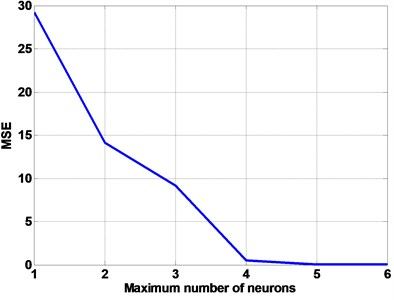Fig. 4Training performance of suggested FFNN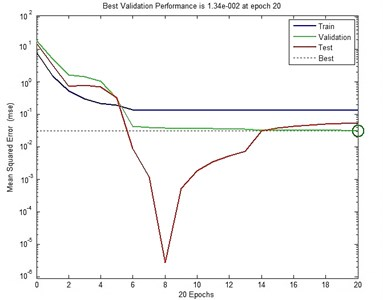Fig. 5Comparison between the FSTM data and FFNN predicted data for Ω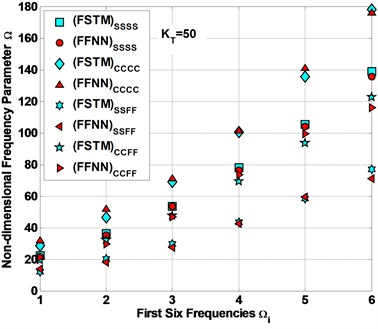Fig. 6Comparison between the FSTM data and FFNN expected data for Ω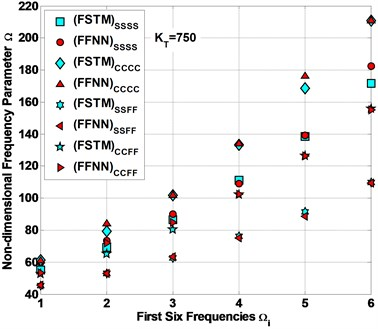Fig. 7The performances of the present FFNN to predict data of Ω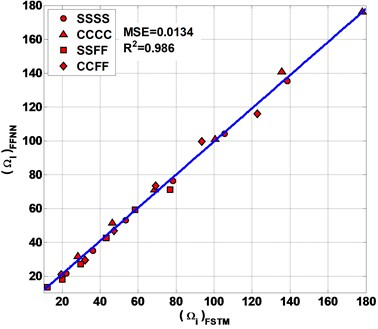Fig. 8The performances of the present FFNN to expect data of Ω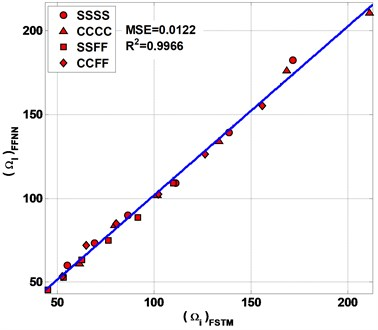Table 3Mean square error (MSE) and regression coefficient (R2) values

 Data MSE ${R}^{2}$ Predicted 0.0134 0.986 Expected 0.0122 0.9966

Table 3 and Figs. 7 and 8 represent the performances of the present FFNN by calculating the values of MSE and ${R}^{2}$ (see Eqs. (5, 6)) between FSTM data for both FFNN predicted and expected data for $\mathrm{\Omega }$. As shown in Fig. 7 the value of MSE and ${R}^{2}$ between the FSTM and FFNN predicted data are 0.0134 and 0.986 respectively. From Fig. 8 the value of MSE and ${R}^{2}$ between the FSTM and FFNN expected data are 0.0122 and 0.9966 respectively.

### 4.4. The use of present FFNN for predicting non-FSTM data of non-dimensional frequency parameter $\mathbf{\Omega }$

The main goal of the artificial neural network design is predicting non- FSTM data. In this section we will use the suggested FFNN to predict some non- FSTM data not included in FSTM evaluation. It is selected to use seven different ${K}_{T}$, for four types of CBCs (SSSS, CCCC, SSFF and CCFF). The previous parameters ${K}_{T}$, $\theta$ are the input vectors for artificial neural network, while the output is the signal vector is $\mathrm{\Omega }$.

Fig. 9The FFNN predicted non-FSTM results of non-dimensional frequency parameter Ω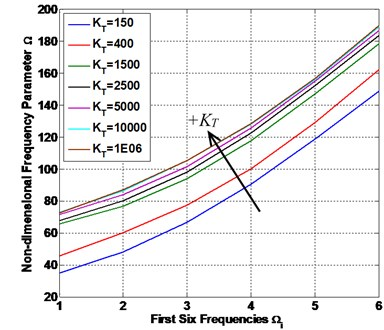a) SSSS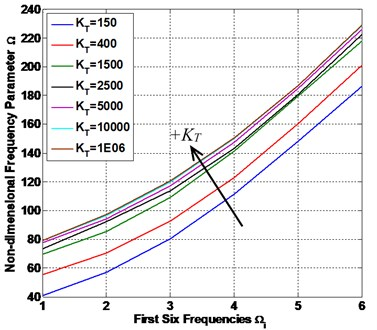b) CCCC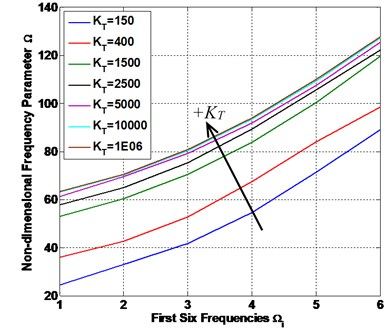c) SSFF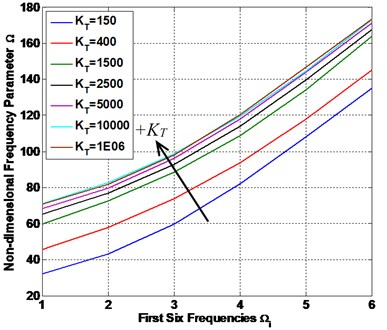d) CCFF

Table 4The predicted non-FSTM results of the first six frequencies by FFNN

 ${K}_{T}$ ${\mathrm{\Omega }}_{1}$ ${\mathrm{\Omega }}_{2}$ ${\mathrm{\Omega }}_{3}$ ${\mathrm{\Omega }}_{4}$ ${\mathrm{\Omega }}_{5}$ ${\mathrm{\Omega }}_{6}$ SSSS 150 Ref  34.6580 48.7340 66.1000 90.7490 118.1000 151.2100 FFNN 34.8971 48.1343 66.6159 90.5891 118.8782 148.7286 400 Ref  45.8453 59.9213 77.2873 101.9363 129.2873 162.3973 FFNN 45.8453 60.1268 77.2873 100.2624 129.2873 162.3973 1500 Ref  62.4709 76.5469 93.9129 118.5619 145.9129 179.0229 FFNN 65.5428 76.7674 93.799 117.6289 147.1225 178.4941 2500 Ref  67.5270 81.6030 98.9690 123.6180 150.9690 184.0790 FFNN 67.6715 80.0752 98.248 122.7383 152.2729 183.4709 5000 Ref  70.7832 84.8592 102.2252 126.8742 154.2252 187.3352 FFNN 71.5277 83.8535 101.5647 125.5413 154.9449 186.8396 10000 Ref  72.7065 86.7825 104.1485 128.7975 156.1485 189.2585 FFNN 72.8107 86.2942 105.2392 128.2564 156.1388 189.2703 1E+06 Ref  73.1195 87.1955 104.5615 129.2105 156.5615 189.6715 FFNN 72.6947 87.0396 105.4209 128.5854 156.8348 189.6596 CCCC 150 Ref  40.9440 59.0155 81.3109 112.9567 148.0714 190.5798 FFNN 41.0549 57.0992 80.3422 111.2997 148.2179 186.4952 400 Ref  52.1313 70.2028 92.4983 124.1440 159.2587 201.7672 FFNN 55.4754 70.442 92.6468 123.0928 160.6131 201.1848 1500 Ref  68.7569 86.8284 109.1239 140.7696 175.8843 218.3928 FFNN 69.7659 85.3865 109.1148 141.4497 179.6854 218.0006 2500 Ref  73.8130 91.8845 114.1800 145.8257 180.9404 223.4489 FFNN 73.4808 92.2119 113.7449 143.3314 180.9021 222.5692 5000 Ref  77.0692 95.1407 117.4361 149.0819 184.1966 226.7050 FFNN 77.8273 94.092 117.0825 147.6263 184.9599 226.1968 10000 Ref  78.9925 97.0640 119.3594 151.0052 186.1199 228.6283 FFNN 79.3118 96.3864 119.7441 150.1071 187.0576 228.5796 1E+06 Ref  79.4055 97.4770 119.7724 151.4182 186.5329 229.0413 FFNN 79.1422 97.2499 120.7791 150.5119 186.7872 229.1948 SSFF 150 Ref  24.7880 32.5903 42.2163 55.8793 71.0400 89.3929 FFNN 24.4094 32.9047 41.7941 54.6268 71.2814 89.0171 400 Ref  35.9753 43.7777 53.4036 67.0666 82.2273 100.5802 FFNN 35.9713 42.5469 52.8278 67.4484 83.9108 98.563 1500 Ref  52.6009 60.4033 70.0293 83.6922 98.8529 117.2058 FFNN 52.904 60.2689 70.3874 83.7121 100.3213 119.6639 2500 Ref  57.6570 65.4594 75.0853 88.7483 103.9090 122.2619 FFNN 57.9028 64.964 75.361 89.2589 105.6161 122.2164 5000 Ref  60.9132 68.7155 78.3415 92.0045 107.1652 125.5181 FFNN 61.3157 69.5938 79.1805 91.7539 107.5848 125.4066 10000 Ref  62.8365 70.6388 80.2648 93.9278 109.0885 127.4414 FFNN 63.0778 70.2994 80.2805 93.3565 109.3353 127.2809 1E+06 Ref  63.2495 71.0518 80.6778 94.3408 109.5015 127.8544 FFNN 63.3054 70.5596 80.69 93.9744 110.0274 127.6158 CCFF 150 Ref  32.1058 44.5596 59.9242 81.7324 105.9312 135.2253 FFNN 32.1827 43.2269 59.686 81.9174 108.2676 135.0946 400 Ref  43.2931 55.7469 71.1115 92.9197 117.1185 146.4127 FFNN 45.562 57.8813 73.8431 93.8403 117.8631 145.3086 1500 Ref  59.9187 72.3725 87.7371 109.5453 133.7442 163.0383 FFNN 59.6275 72.4773 88.4423 108.7844 134.0482 163.8665 2500 Ref  64.9748 77.4286 92.7932 114.6014 138.8002 168.0944 FFNN 65.0645 76.7108 92.8809 114.0148 139.5554 167.6554 5000 Ref  68.2310 80.6848 96.0494 117.8576 142.0564 171.3505 FFNN 68.3337 79.7057 96.0889 117.8545 143.8554 171.2413 10000 Ref  70.1543 82.6081 97.9727 119.7809 143.9797 173.2738 FFNN 70.9017 82.7021 98.6556 119.3321 144.5419 173.0477 1E+06 Ref  70.5673 83.0211 98.3857 120.1939 144.3927 173.6868 FFNN 70.6424 81.6968 98.1541 120.3744 146.7246 173.5624

Fig. 10The performances of the present FFNN to predicted non-FSTM results of non-dimensional frequency parameter Ω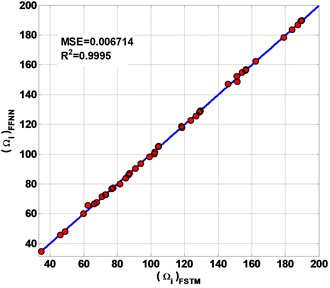a) SSSS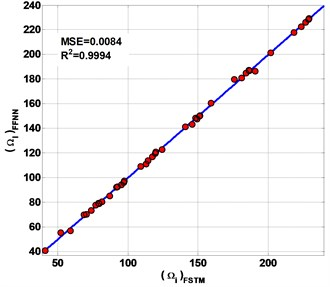b) CCCC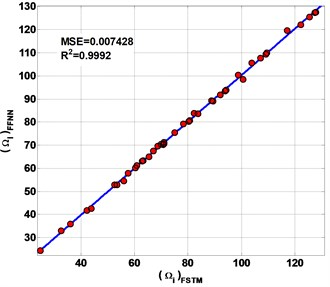c) SSFF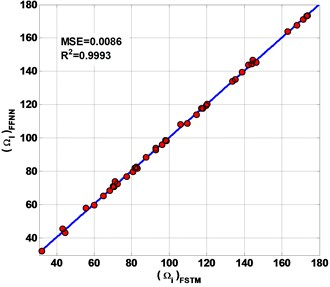d) CCFF

Fig. 9 shows the FFNN predicted results of the first six frequencies of the laminated composite plate are presented in this study. The plate has $\beta =$0.5 and $\mathrm{\Delta }=$0.5 with four different types of CBCs.

The predicted non-FSTM results of the first six frequencies by FFNN and a comparison with the previous work results in literature of FSTM method by Altabey  are presented in Table 4. As a result, a FFNN gave good prediction for non-FSTM data even for extrapolations $\mathrm{\Omega }$ in presented laminated composite plate.

From Table 4 and Fig. 9, we can see, the first six frequencies increase with the increasing of the value of ${K}_{T}$ and we are observed that the gap between values of frequencies in the small ${K}_{T}$ and next values of ${K}_{T}$ are higher than the gap between values of frequencies in the large ${K}_{T}$, and the frequencies at high values of ${K}_{T}$ are almost constant, for all conditions (SSSS, CCCC, SSFF and CCFF). On the other hand, the fully clamped (CCCC) and semi-simply supported (SSFF) condition have the higher and lower values of frequencies respectively and the other two conditions (SSSS) and (CCFF) are lie between them with an intermediate value. This conclusion was found in other work .

${R}^{2}$ of non-FSTM results are 0.9995, 0.9994, 0.9992 and 0.9993 for SSSS, CCCC, SSFF and CCFF plate respectively. All of the predicting results are plotted on the diagonal line (Fig. 10) to observe the performances of the present FFNN to predict non-FSTM of $\mathrm{\Omega }$. The MSE is defined of the predicted non- FSTM. The MSE is of non-FSTM results are 0.006714, 0.0084, 0.007428 and 0.0086 for SSSS, CCCC, SSFF and CCFF plate respectively.

## 5. Conclusions

The natural frequency values were found by analyses which were done of basalt FRP laminated variable thickness rectangular plates with IES by FSTM method and ANNs algorithm, which are combined to decrease computational effort to discern natural frequency in basalt FRP laminated composite plates, in order to save the time of the FSTM computational data to a minimum with high accuracy and easy. Based on the FSTM results and the results predicted by artificial neural networks, the following conclusions are drawn for laminated composite material plates.

1) The FFNN model has been developed by considering the ${K}_{T}$ and ply angle ($\theta$) as the input for predicting $\mathrm{\Omega }$. The developed FFNN model could predict $\mathrm{\Omega }$ with the ${R}^{2}$ and MSE are 0.986 and 0.0134 for training data set and 0.9966 and 0.0122 for test data respectively.

2) The FFNN model could predict non-FSTM of $\mathrm{\Omega }$ with ${R}^{2}$ of non-FSTM results are 0.9995, 0.9994, 0.9992 and 0.9993 for SSSS, CCCC, SSFF and CCFF plate respectively. The MSE is defined of the predicted non-FSTM. The MSE is of non-FSTM results are 0.006714, 0.0084, 0.007428 and 0.0086 for SSSS, CCCC, SSFF and CCFF plate respectively.

3) The ANN predicted results are in very good agreement with the FSTM results.

#### Cited by

Proceedings of the 4th International Conference on Advances in Civil and Ecological Engineering Research
Proceedings of the 4th International Conference on Advances in Civil and Ecological Engineering Research
Third International Conference on Computer Vision and Information Technology (CVIT 2022)
Experimental and Computational Analysis of Free In-Plane Vibration of Curved Beams
2022 International Conference on Electrical, Computer, Communications and Mechatronics Engineering (ICECCME)
2022 International Conference on Electrical, Computer, Communications and Mechatronics Engineering (ICECCME)
Proceedings of the 4th International Conference on Intelligent Science and Technology
Deep Learning-Based Crack Identification for Steel Pipelines by Extracting Features from 3D Shadow Modeling